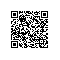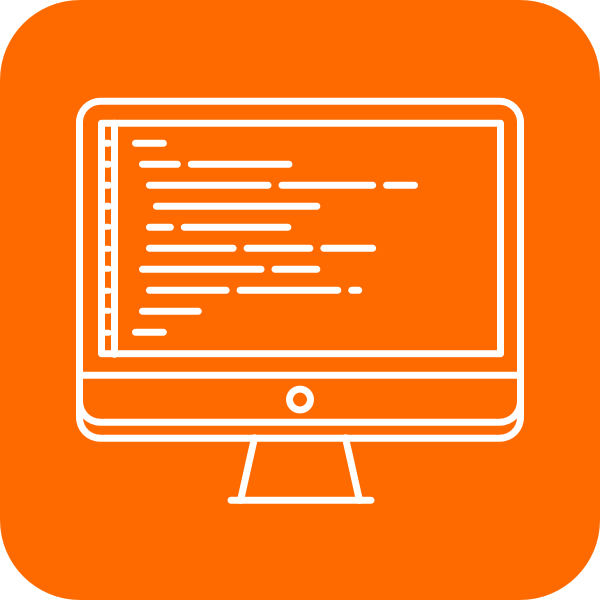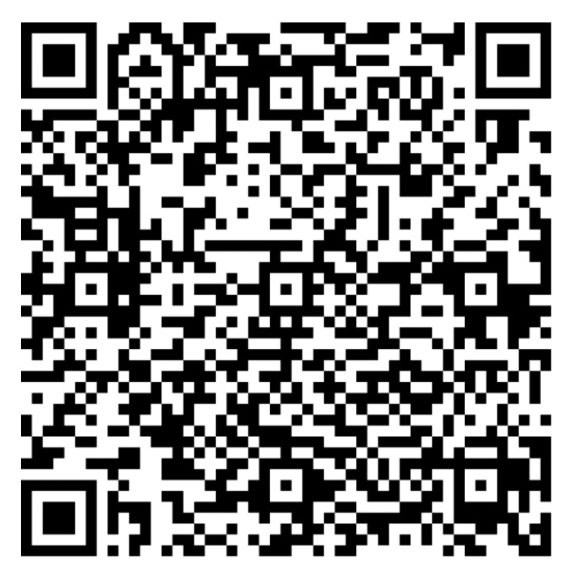# 大厂面试真题详解：字符串解码

输入: S = abc3[a]

输入: S = 3[2[ad]3[pf]]xyz

### 题解

class Solution:
"""
@param s: an expression includes numbers, letters and brackets
@return: a string
"""
def expressionExpand(self, s):
stack = []
for c in s:
if c != ']':
stack.append(c)
continue

strs = []
while stack and stack[-1] != '[':
strs.append(stack.pop())

# skip '['
stack.pop()

repeats = 0
base = 1
while stack and stack[-1].isdigit():
repeats += (ord(stack.pop()) - ord('0')) * base
base *= 10
stack.append(''.join(reversed(strs)) * repeats)

return ''.join(stack)使用钉钉扫一扫加入圈子
+ 订阅